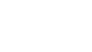# Solutions

Get detailed explanations to advanced GMAT questions.

### QuestionThe logo of a certain corporation is in the shape of a polygon, where all angles of a the polygon have equal measures. In the above figure V is one vertex of the polygon. If Y in degrees is the measure of an angle of the polygon and y=5x how many sides does the polygon have?

Option A:

5

Option B:

6

Option C:

10

Option D:

12

Option E:

30

Hard

### Solution

Option D is the correct answer.

### Option Analysis

From the figure,

x + y = 180

6*x = 180

x = 30, y = 150

Let number of sides be “n”, hence number of interior angles is also “n”

Sum of all interior angles = 150*n

Formula for sum of interior angles

= 180*(n-2) 180*(n-2) = 150*n

30*n = 360

n = 360/30 = 12